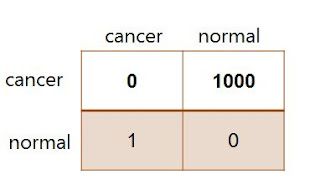# How to Reduce Expected Loss in Classification (Machine Learning)

This lessons explains in simple terms how to minimize expected loss during classification.
Remember that when an input variable is classified wrongly, a loss is incurred. However, this loss can be large or can be minimal

Example of Cancer
Scenario 1: A patient that does not have cancer is misclassified as having cancer
Scenario 2: A patient that has cancer is misclassified as not having cancer.

For these two misclassification scenarios, we can see that the loss incurred by the second scenario can be enormous, which may actually be death of the patient.

So the objective is to reduce such misclassifications that would result in much loss. That means reducing the expected loss

Formalism
We can formalize the discussion by introducing a loss function also known as cost function. The loss function is a single overall measure of the  loss incurred in taking any of the available decisions.

The goal is to minimize this total loss incurred.

The Loss Matrix
The Loss Matrix is a table showing the decision that was taken relative to the true class. This is shown in Figure 1:Figure 1: Loss Matrix for the Cancer Example

The elements of the  loss matrix represents the loss incurred by taking one of the available decision. The rows of the loss matrix represents the true class while the columns of the matrix represents the assignment of class based on our decision

Assuming that for a new value of x, we assign it to class Cj whereas the real correct class is Ck. It means we have incurred a loss Lkj, which is the k, j element of the loss matrix.
The average loss function is given by the equation:

The best solution is one that minimizes the average loss function. For a given input vector x, our uncertainty in the correct class is expressed through the joint probability distribution p(x, Ck).

As before, we can use the product rule which states that

p(x, Ck) = p(Ck | x)p(x)

to eliminate the common factor p(x). Therefore, the decision rule that minimizes the expected loss is one that assigns x to the class for which the quantity:

is minimum.
This can be found once we know the posterior class probabilities p(Ck | x)